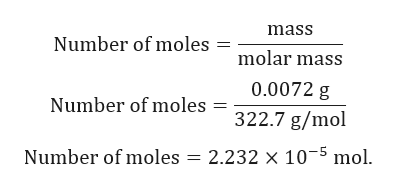# Cyanidin chloride (C15H11O6Cl) contains the cyanidin ion, a pigment found in many berries. Calculate the number of miles of cyanidin chloride equivalent to 7.2 mg.

Question
37 views

Cyanidin chloride (C15H11O6Cl) contains the cyanidin ion, a pigment found in many berries. Calculate the number of miles of cyanidin chloride equivalent to 7.2 mg.

check_circle

Step 1

Here, we have to calculate number of moles of cyanidin chloride present in 7.2 mg.

Step 2

Given:

Mass of cyanidin chloride = 7.2 mg = 0.0072 g.

Molar mass of cyanidin chloride = 322.7 g/mol.

Step 3

Calculation for number of mole...help_outlineImage Transcriptionclosemass Number of moles molar mass 0.0072 g Number of moles=322.7 g/mol Number of moles = 2.232 x 105 mol fullscreen

### Want to see the full answer?

See Solution

#### Want to see this answer and more?

Solutions are written by subject experts who are available 24/7. Questions are typically answered within 1 hour.*

See Solution
*Response times may vary by subject and question.
Tagged in

### General Chemistry